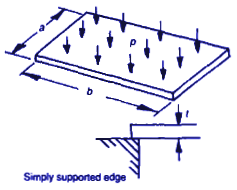Related Resources: material science

### Rectangular Plate Uniform Load Simply Supported Equations and Calculator

Rectangular plate, uniform load, simply supported (Empirical) equations and calculator

Since comers tend to rise off the supports, vertical movement must be prevented without restricting rotation.

Symbols used:
a = minor length of rectangular plate, (m, in)
b = major length of rectangular plate, (m, in)
v = Poisson’s ratio
E = Young’s modulus, (N/m2, lbs/in2)
t = plate thickness, (m, in)
s m = maximum stress, (N/m2, lbs/in2)
ym = maximum deflection, (m, in)Stress At CenterDeflection at centerD = flexural rigidity= Et3/(12 ( 1 -v2)